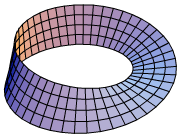# Configuration space

"C-space" redirects here. For the art gallery, see C-Space, Beijing.
For the computer hardware concept, see PCI configuration space.

In classical mechanics, the parameters that define the configuration of a system are called generalized coordinates, and the vector space defined by these coordinates is called the configuration space of the physical system. It is often the case that these parameters satisfy mathematical constraints, such that the set of actual configurations of the system is a manifold in the space of generalized coordinates. This manifold is called the configuration manifold of the system.

## Configuration spaces in physics

### Particle

The position of a single particle moving in ordinary Euclidean 3-space is defined by the vector r=(x, y, z), and therefore its configuration space is R3. If the particle is constrained to lie on a sphere, then its configuration space is the subset of coordinates in R3 that define points on the sphere S2.

For n particles the configuration space is R3n, or possibly the subspace where no two positions are equal.

An important problem in physics considers the set of all trajectories of a particle between two points, which is a configuration space that is also known as a function space M. In quantum mechanics one formulation uses histories, or trajectories, as configurations.

### Rigid body

The set of coordinates that define the position of a reference point and the orientation of a coordinate frame attached to a rigid body in three-dimensional space form its configuration space, often denotedwhererepresents the coordinates of the origin of the frame attached to the body, andrepresents the rotation matrices that define the orientation of this frame relative to a ground frame. A configuration of the rigid body is defined by six parameters, three fromand three from, and is said to have six degrees of freedom.

In Robotics, configuration space refers to the set of reachable positions by a robot's end-effector, considered as a rigid body in three-dimensional space. Thus positions of the end-effector of a robot can be identified with the group of spatial rigid transformations, often denoted SE(3).

The joint parameters of the robot are used as generalized coordinates to define configurations. The set of joint parameter values is called the joint space. A robot's forward and inverse kinematics equations define maps between configurations and end-effector positions, or between joint space and configuration space. Robot motion planning uses this mapping to find a path in joint space that provides an achievable route in the configuration space of the end-effector.

### Phase space

In Mechanics, the configuration of a system consists of the positions had by all components subject to kinematical constraints. The set of velocities available to a system defines a plane tangent to the configuration manifold of the system. Momentum vectors are linear functionals of the tangent plane, known as cotangent vectors. Thus, the set of positions and momenta of a mechanical system forms the cotangent bundle of the configuration manifold.

This larger manifold is called the phase space of the system. The joint space and configuration space can be viewed as a bijection on the phase space of a mechanical system.

## Configuration spaces in mathematicsThe configuration space of all unordered pairs of distinct points on the circle is the Möbius strip.

In mathematics a configuration space refers to a broad family of constructions closely related to the state space notion in physics. The most common notion of configuration space in mathematicsis the set of n-element subsets of a topological space. This set is given a topology by considering it as the quotientwhereandis the symmetric group acting by permuting the coordinates of. Typically,is called the configuration space of n unordered points inandis called the configuration space of n ordered or colored points in; the space of n ordered not necessarily distinct points is simplyIf the original space is a manifold, the configuration space of distinct, unordered points is also a manifold, while the configuration space of not necessarily distinct unordered points is instead an orbifold.

Configuration spaces are related to braid theory, where the braid group is considered as the fundamental group of the space.

A configuration space is a type of classifying space or (fine) moduli space. In particular, there is a universal bundlewhich is a sub-bundle of the trivial bundle, and which has the property that the fiber over each pointis the n element subset ofclassified by p.

The homotopy type of configuration spaces is not homotopy invariant – for example, the spacesare not homotopy equivalent for any two distinct values of. For instance,is not connected,is a, andis simply connected for.

It used to be an open question whether there were examples of compact manifolds which were homotopy equivalent but had non-homotopy equivalent configuration spaces: such an example was found only in 2005 by Longoni and Salvatore. Their example are two three-dimensional lens spaces, and the configuration spaces of at least two points in them. That these configuration spaces are not homotopy equivalent was detected by Massey products in their respective universal covers.